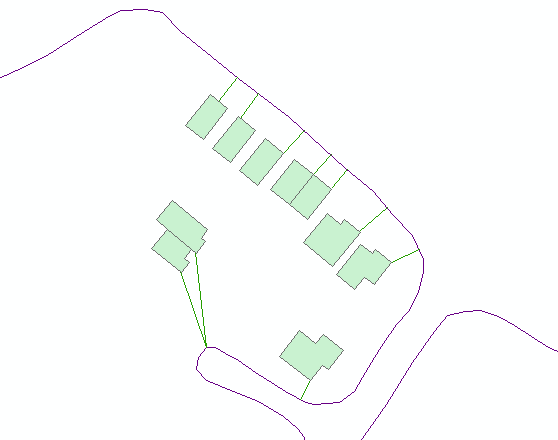# Generate Near Table

6139
13
02-20-2015 08:48 AMNew Contributor III

Hi Guys,

hope everyone is well?

I'm hoping someone can help me here, is there a way to generate something like a 'near table' (http://help.arcgis.com/en/arcgisdesktop/10.0/help/index.html#//00080000001n000000) with a basic level user licence?

Are there any work around's you can suggest?

Thanks in advance for any help.

I'm using ArcGIS 10.2

Tags (3)
1 Solution

Accepted SolutionsbyEsri Esteemed Contributor

I normally run the code from a stand alone IDE (I use PyScripter), but you can run the code from the python window (it will not add the result to the TOC, though). Before you run it, make sure you change the paths to your input data and provide a valid output name/path.

You can also create a toolbox from it. Use the arcpy.GetParameterAsText()  to collect the inputs

```    fc_pol = arcpy.GetParameterAsText(0) # featurelayer, filter on polygon, direction is input
fc_lin = arcpy.GetParameterAsText(1) # featurelayer, filter on polyline, direction is input
fc_out = arcpy.GetParameterAsText(2) # featureclass, direction is output```

If you have problems, post them back and I can have a look at it.

13 RepliesbyEsri Esteemed Contributor

You can probably do something (very) simplified with some Python code, loading the data into a dictionary and analyzing it, but this method should not be executed on larger data sets or multiple data sets. How many featureclasses with how many features and what geometry type do you want to use?New Contributor III

Hi Xander,

I have 1000s of points, in this case just under 5000.

Additionally, I wouldnt have a clue where to begin with pythonbyEsri Esteemed Contributor

Are the points in a single featureclass or are they stored in 2 or more featureclasses. I could probably write something, but it depends on what you want to achieve.

What options should be supported:

• location
• angle
• closest
• closest_count
• method

From all the development languages for ArcGIS, Python is probably the easiest to learn...New Contributor III

Hi Xander,

What I did was convert a polygon to a points, what I was hoping to achieve is get the xy of of the closest point on a polygon to the closest point on a polyline (which I also converted to points, so I could get the xy). In the end my aim is to create a line between the 2 XY points, for this I would have used XY to Line tool.

Thanks again,byEsri Esteemed Contributor

OK, that sounds a little different, but this can be done using some Python code. What I wonder is how the polygon was translated to point. Did you use the label point or true centroid or is it a point on the perimeter of the polygon?

The line does not have to be converted to points. For each point (from the polygon) to nearest point to a line can be determined. This can yield to point on the line too, which subsequently can be used to create the "near" line.New Contributor III

Hi Xander, apologies for the late reply.

I converted the polygon to lines, which was straight forward and then created points along the line using a toolbox called Create Points On Line (http://ianbroad.com/arcgis-toolbox-create-points-polylines-arcpy/). Thinking about it now it clearly wasn't the right approach.byEsri Esteemed Contributor

The idea of converting the polygon and line features to points and perform the analysis with the points is not a strange or bad idea. Just look at this thread: Distance between two linear features?  which does the same (yet requires an Advanced/ArcInfo license)

Just to understand a little more of what you are doing, what do the polygons and lines represent? Can you attach a screendump of your data? This to determine the complexity of your geometries.

What I would be thinking of is using Python to loop through points on the boundary of the polygon (no conversion to lines or points needed) and use the Polyline::queryPointAndDistance (in_point, {as_percentage}) method to query the nearest point on the line feature.

From the Help:

Finds the point on the polyline nearest to the in_point and the distance between those points. Also returns information about the side of the line the in_point is on as well as the distance along the line where the nearest point occurs.

If possible you could attach a small sample of the data you have, and I'll write some code which you can use to process your data.New Contributor III

Hi Xander, The Polygons represent homes and the polylines are road bounderies. What Im trying to go is show a line from the road boundary to the home boundary but using the shortest line.

I haven't attached a screen dump of what I want but I have attached my shapefiles. Hope this helps? Let me know i there is anything additional you may need.

Regards

SukhbyEsri Esteemed Contributor

The code below will generate the following result:Change the input and output featureclasses on line 5, 6 and 7. The output featureclass (shapefile) will be created.

On line 10 you can play with the precision (stepsize). In this case I specified 1 (m).

```def main():
import arcpy

# inputs and output
fc_pol = r"D:\Xander\GeoNet\NearLines\Polygons.shp"
fc_out = r"D:\Xander\GeoNet\NearLines\lines02.shp"

# precision (m)
stepsize = 1 # extract point on boundary polygon every 1 meter

sr = arcpy.Describe(fc_lin).spatialReference

# create list of line geometries
lst_lines = [r for r in arcpy.da.SearchCursor(fc_lin, ("SHAPE@"))]

# loop through polygons
lst_res = []
with arcpy.da.SearchCursor(fc_pol, ("SHAPE@")) as curs:
for row in curs:
polygon = row
boundary = polygon.boundary()
dist_min = None

for i in range(0, int(boundary.length), stepsize):
point_pol = boundary.positionAlongLine(i, False)

for line in lst_lines:
pntg, val, dist, right_side = line.queryPointAndDistance(point_pol, False)
if dist_min is None:
dist_min = dist
pnt_lin_found = arcpy.Point(pntg.firstPoint.X, pntg.firstPoint.Y)
pnt_pol_found = arcpy.Point(point_pol.firstPoint.X, point_pol.firstPoint.Y)
else:
if dist < dist_min:
dist_min = dist
pnt_lin_found = arcpy.Point(pntg.firstPoint.X, pntg.firstPoint.Y)
pnt_pol_found = arcpy.Point(point_pol.firstPoint.X, point_pol.firstPoint.Y)

line_out = arcpy.Polyline(arcpy.Array([pnt_lin_found, pnt_pol_found]), sr)
lst_res.append(line_out)

arcpy.CopyFeatures_management(lst_res, fc_out)

if __name__ == '__main__':
main()```

Kind regards, Xander